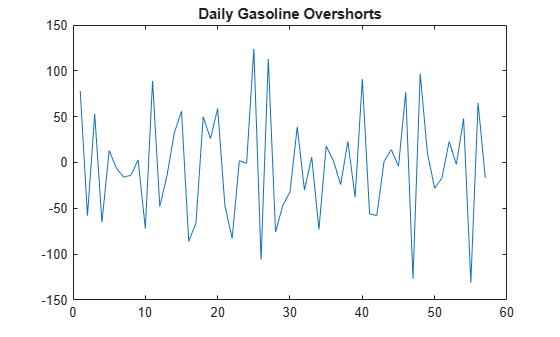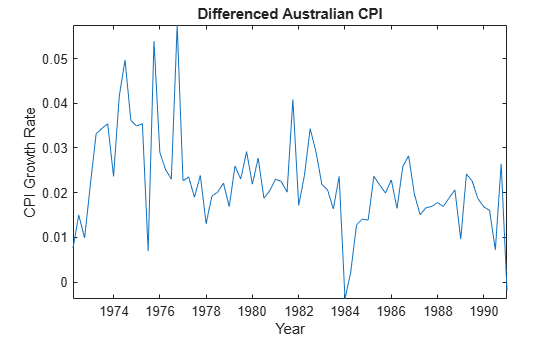lbqtest

Ljung-Box Q-test for residual autocorrelation

Description

example

h = lbqtest(res) returns a logical value (h) with the rejection decision from conducting a Ljung-Box Q-Test for autocorrelation in the residual series res.

example

h = lbqtest(res,Name,Value) uses additional options specified by one or more name-value pair arguments.

• If any name-value pair argument is a vector, then all name-value pair arguments specified must be vectors of equal length or length one. lbqtest(res,Name,Value) treats each element of a vector input as a separate test, and returns a vector of rejection decisions.

• If any name-value pair argument is a row vector, then lbqtest(res,Name,Value) returns a row vector.

example

[h,pValue] = lbqtest(___) returns the rejection decision and p-value for the hypothesis test, using any of the input arguments in the previous syntaxes.

example

[h,pValue,stat,cValue] = lbqtest(___) additionally returns the test statistic (stat) and critical value (cValue) for the hypothesis test.

Examples

collapse all

Load the Deutschmark/British pound foreign-exchange rate data set.

Convert the prices to returns.

returns = price2ret(Data);

Compute the deviations of the return series.

res = returns - mean(returns);

Test the hypothesis that the residual series is not autocorrelated, using the default number of lags.

h1 = lbqtest(res)
h1 = logical
0

h1 = 0 indicates that there is not enough evidence to reject the null hypothesis that the residuals of the returns are not autocorrelated.

Test the hypothesis that there are significant ARCH effects, using the default number of lags .

h2 = lbqtest(res.^2)
h2 = logical
1

h2 = 1 indicates that there are significant ARCH effects in the residuals of the returns.

Test for residual heteroscedasticity using archtest and the default number of lags.

h3 = archtest(res)
h3 = logical
1

h3 = 1 indicates that the null hypothesis of no residual heteroscedasticity should be rejected in favor of an ARCH(1) model. This result is consistent with h2.

Conduct multiple Ljung-Box Q-tests for autocorrelation by including various lags in the test statistic. The data set is a time series of 57 consecutive days of overshorts from an underground gasoline tank in Colorado . That is, the current overshort (${y}_{t}$) represents the accuracy in measuring the amount of fuel:

• In the tank at the end of day $t$

• In the tank at the end of day $t-1$

• Delivered to the tank on day $t$

• Sold on day $t$.

y = Data;
T = length(y); % Sample size

figure
plot(y)
title('Overshorts for 57 Consecutive Days')lbqtest is appropriate for a series with a constant mean. Since the series appears to fluctuate around a constant mean, you do not need to transform the data.

Compute the residuals.

res = y - mean(y);

Assess whether the residuals are autocorrelated. Include 5, 10, and 15 lags in the test statistic computation.

[h,pValue] = lbqtest(res,'lags',[5,10,15])
h = 1x3 logical array

1   1   1

pValue = 1×3

0.0016    0.0007    0.0013

h and pValue are vectors containing three elements corresponding to tests at each of the three lags. The first element of each output corresponds to the test at lag 5, the second element corresponds to the test at lag 10, and the third element corresponds to the test at lag 15.

h = 1 indicates the rejection of the null hypothesis that the residuals are not autocorrelated. pValue indicates the strength at which the test rejects the null hypothesis. Since all three $p-values$ are less than 0.01, there is strong evidence to reject the null hypothesis that the residuals are not autocorrelated.

Infer residuals from an estimated ARIMA model, and assess whether the residuals exhibit autocorrelation using lbqtest.

Load the Australian Consumer Price Index (CPI) data set. The time series (cpi) is the log quarterly CPI from 1972 to 1991. Remove the trend in the series by taking the first difference.

cpi = DataTable.PAU;
T = length(cpi);
dCPI = diff(cpi);

figure
plot(dates(2:T),dCPI)
title('Differenced Australian CPI')
xlabel('Year')
ylabel('CPI growth rate')
datetick
axis tightThe differenced series appears stationary.

Fit an AR(1) model to the series, and then infer residuals from the estimated model.

Mdl = arima(1,0,0);
EstMdl = estimate(Mdl,dCPI);

ARIMA(1,0,0) Model (Gaussian Distribution):

Value      StandardError    TStatistic      PValue
_________    _____________    __________    __________

Constant     0.015564      0.0028766        5.4106      6.2808e-08
AR{1}         0.29646        0.11048        2.6834       0.0072876
Variance    0.0001038     1.1932e-05        8.6994      3.3362e-18
res = infer(EstMdl,dCPI);
stdRes = res/sqrt(EstMdl.Variance); % Standardized residuals

Assess whether the residuals are autocorrelated by conducting a Ljung-Box Q-test. The standardized residuals originate from the estimated model (EstMdl) containing parameters. When using such residuals, it is best practice to do the following:

• Adjust the degrees of freedom (dof) of the test statistic distribution to account for the estimated parameters.

• Set the number of lags to include in the test statistic.

• When you count the estimated parameters, skip the constant and variance parameters.

lags = 10;
dof  = lags - 1; % One autoregressive parameter

[h,pValue] = lbqtest(stdRes,'Lags',lags,'DOF',dof)
h = logical
1

pValue = 0.0119

pValue = 0.0130 suggests that there is significant autocorrelation in the residuals at the 5% level.

Input Arguments

collapse all

Residual series for which the software computes the test statistic, specified as a numeric vector. The last element corresponds to the latest observation.

Typically, you fit a model to an observed time series, and res contains the standardized residuals from the fitted model.

Specify missing observations using NaN. The lbqtest function treats missing values as missing completely at random.

Data Types: double

Name-Value Arguments

Specify optional comma-separated pairs of Name,Value arguments. Name is the argument name and Value is the corresponding value. Name must appear inside quotes. You can specify several name and value pair arguments in any order as Name1,Value1,...,NameN,ValueN.

Example: 'Lags',1:4,'Alpha',0.1 specifies four tests with 1, 2, 3, and 4 lagged terms conducted at the 0.1 significance level.

Number of lagged terms to include in the test statistic calculation, specified as the comma-separated pair consisting of 'Lags' and a positive integer or vector of positive integers.

Use a vector to conduct multiple tests.

Each element of Lags must be less than T, which is the effective sample size of res (that is, the number of nonmissing values in res).

Example: 'Lags',1:4

Data Types: double

Significance levels for the hypothesis tests, specified as the comma-separated pair consisting of 'Alpha' and a scalar or vector.

Use a vector to conduct multiple tests.

Each element of Alpha must be greater than 0 and less than 1.

Example: 'Alpha',0.01

Data Types: double

Degrees of freedom for the asymptotic, chi-square distribution of the test statistics, specified as the comma-separated pair consisting of 'DoF' and a positive integer or vector of positive integers.

Use a vector to conduct multiple tests.

If DoF is an integer, then it must be less than or equal to Lags. Otherwise, each element of DoF must be less than or equal to the corresponding element of Lags.

Example: 'DoF',15

Data Types: double

Output Arguments

collapse all

Test rejection decisions, returned as a logical value or vector of logical values with a length equal to the number of tests that the software conducts.

• h = 1 indicates rejection of the no residual autocorrelation null hypothesis in favor of the alternative.

• h = 0 indicates failure to reject the no residual autocorrelation null hypothesis.

Test statistic p-values, returned as a scalar or vector with a length equal to the number of tests that the software conducts.

Test statistics, returned as a scalar or vector with a length equal to the number of tests that the software conducts.

Critical values determined by Alpha, returned as a scalar or vector with a length equal to the number of tests that the software conducts.

collapse all

Ljung-Box Q-Test

The Ljung-Box Q-test is a “portmanteau” test that assesses the null hypothesis that a series of residuals exhibits no autocorrelation for a fixed number of lags L, against the alternative that some autocorrelation coefficient ρ(k), k = 1, ..., L, is nonzero.

The test statistic is

$Q=T\left(T+2\right)\sum _{k=1}^{L}\left(\frac{\rho {\left(k\right)}^{2}}{\left(T-k\right)}\right),$

where T is the sample size, L is the number of autocorrelation lags, and ρ(k) is the sample autocorrelation at lag k. Under the null hypothesis, the asymptotic distribution of Q is chi-square with L degrees of freedom.

Missing Completely at Random

Observations of a random variable are missing completely at random if the tendency of an observation to be missing is independent of both the random variable and the tendency of all other observations to be missing.

Tips

If you obtain res by fitting a model to data, then you should reduce the degrees of freedom (the argument DoF) by the number of estimated coefficients, excluding constants. For example, if you obtain res by fitting an ARMA(p,q) model, set DoF to Lpq, where L is Lags.

Algorithms

• The Lags argument affects the power of the test.

• If L is too small, then the test does not detect high-order autocorrelations.

• If L is too large, then the test loses power when a significant correlation at one lag is washed out by insignificant correlations at other lags.

• Box, Jenkins, and Reinsel suggest setting min[20,T-1] as the default value for lags .

• Tsay cites simulation evidence that setting lags to a value approximating log(T) provides better power performance .

• lbqtest does not directly test for serial dependencies other than autocorrelation. However, you can use it to identify conditional heteroscedasticity (ARCH effects) by testing squared residuals .

Engle's test assesses the significance of ARCH effects directly. For details, see archtest.

 Box, G. E. P., G. M. Jenkins, and G. C. Reinsel. Time Series Analysis: Forecasting and Control. 3rd ed. Englewood Cliffs, NJ: Prentice Hall, 1994.

 Brockwell, P. J. and R. A. Davis. Introduction to Time Series and Forecasting. 2nd ed. New York, NY: Springer, 2002.

 Gourieroux, C. ARCH Models and Financial Applications. New York: Springer-Verlag, 1997.

 McLeod, A. I. and W. K. Li. "Diagnostic Checking ARMA Time Series Models Using Squared-Residual Autocorrelations." Journal of Time Series Analysis. Vol. 4, 1983, pp. 269–273.

 Tsay, R. S. Analysis of Financial Time Series. 2nd Ed. Hoboken, NJ: John Wiley & Sons, Inc., 2005.